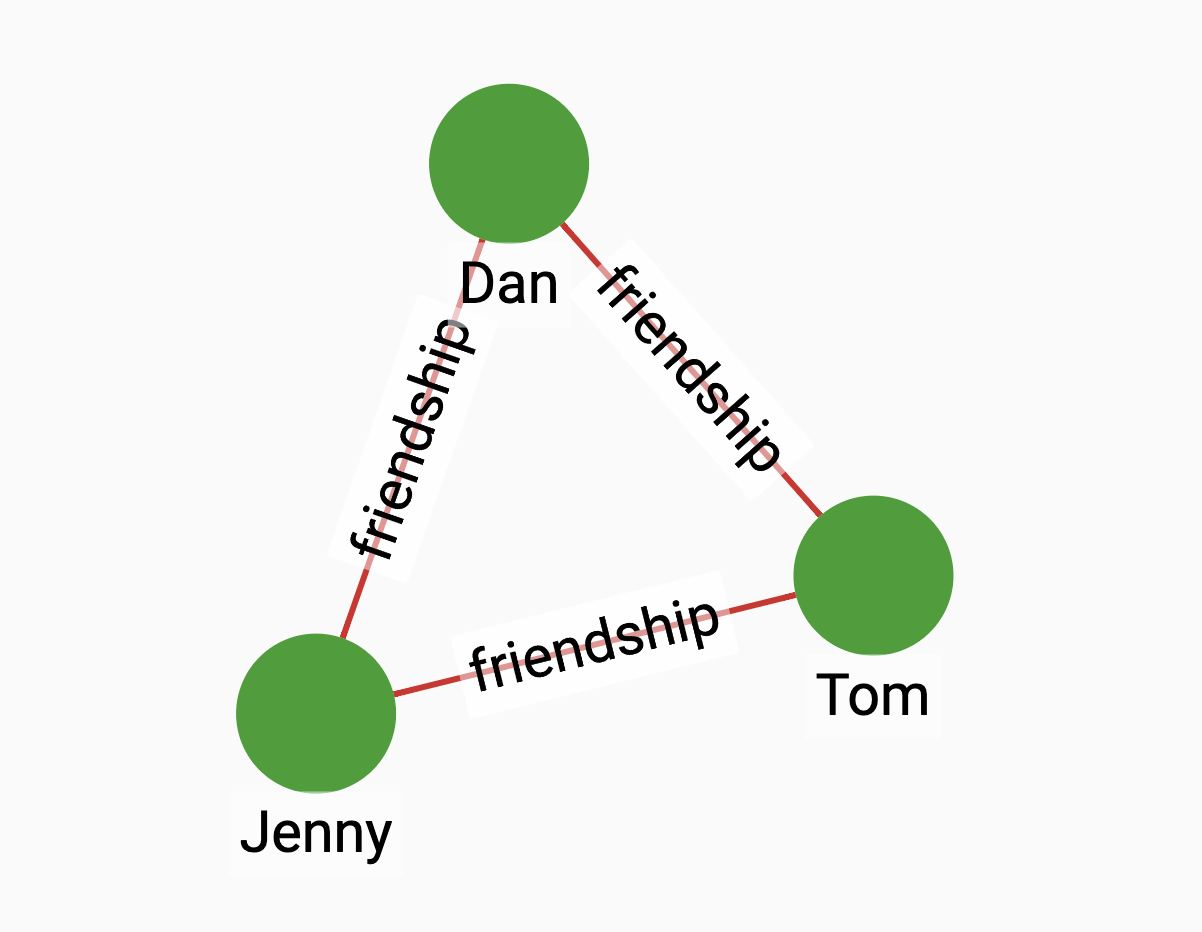# Common Neighbors

The common neighbors algorithm calculates the number of common neighbors between two vertices.

## Specification

``CREATE QUERY tg_common_neighbors(VERTEX a, VERTEX b, SET<STRING> e_type)``

### Time complexity

The algorithm has a time complexity of O(D1 + D2), where D1 and D2 are the degrees of the two vertices.

## Parameters

Name Description Data type

`a`

A vertex.

`VERTEX`

`b`

A vertex.

`VERTEX`

`e_type`

Edge types to traverse.

`SET<STRING>`

## Return value

The number of common neighbors between two vertices.

## Example

Suppose to have the following graph:Running the algorithm between Dan and Jenny will show that they have 1 common neighbor.

• Query

• Result

`` RUN QUERY common_neighbors (("Jenny", "person"), ("Dan", "person"), ["friendship"])``
``````{
"error": false,
"message": "",
"version": {
"schema": 1,
"edition": "enterprise",
"api": "v2"
},
"results": [{"closeness": 1}]
}``````